# Hilbert syzygy theorem

This article gives the statement, and possibly proof, of a commutative unital ring property satisfying a commutative unital ring metaproperty
View all commutative unital ring metaproperty satisfactions | View all commutative unital ring metaproperty dissatisfactions |Get help on looking up metaproperty (dis)satisfactions for commutative unital ring properties
|

## Name

This result is termed the Hilbert syzygy theorem.

## Statement

### Verbal statement

Minimal resolutions over a multivariate polynomial ring over a field of finitely generated graded modules have length bounded by the number of variables.

### Symbolic statement

Let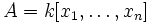$A = k[x_1, \dots, x_n]$ be a polynomial ring over a field$k$ and$M$ a finitely generated graded$A$-module. Then there exists an exact sequence with degree 0 maps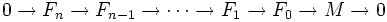$0 \to F_n \to F_{n-1} \to \cdots \to F_1 \to F_0 \to M \to 0$

where the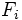$F_i$ are free modules.

## Proof

### Proof idea

The key tools of the proof are the symmetry of Tor, and the Koszul complex.

The idea is that the Koszul complex of$k$ has length$n$ and hence$\mathrm{Tor}^A_i(k,M) = \mathrm{Tor}^A_i(M,k) = 0$ for$i>n$. Using this symmetry, one can also compute the Tor groups by tensoring a free resolution of$M$ by$k$. In particular, taking a minimal free resolution (it is easy to see that minimal free resolutions exist)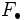$F_\bullet$ of$M$, the differentials become 0 upon tensoring with$k$ by definition of minimal resolution. Hence it follows that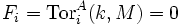$F_i = \mathrm{Tor}^A_i(k,M) = 0$ for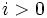$i>0$.

## Remarks

We are assuming that$A$ has the canonical grading, i.e., that the degree$i$ part is the vector space generated by the monomials of degree$i$.